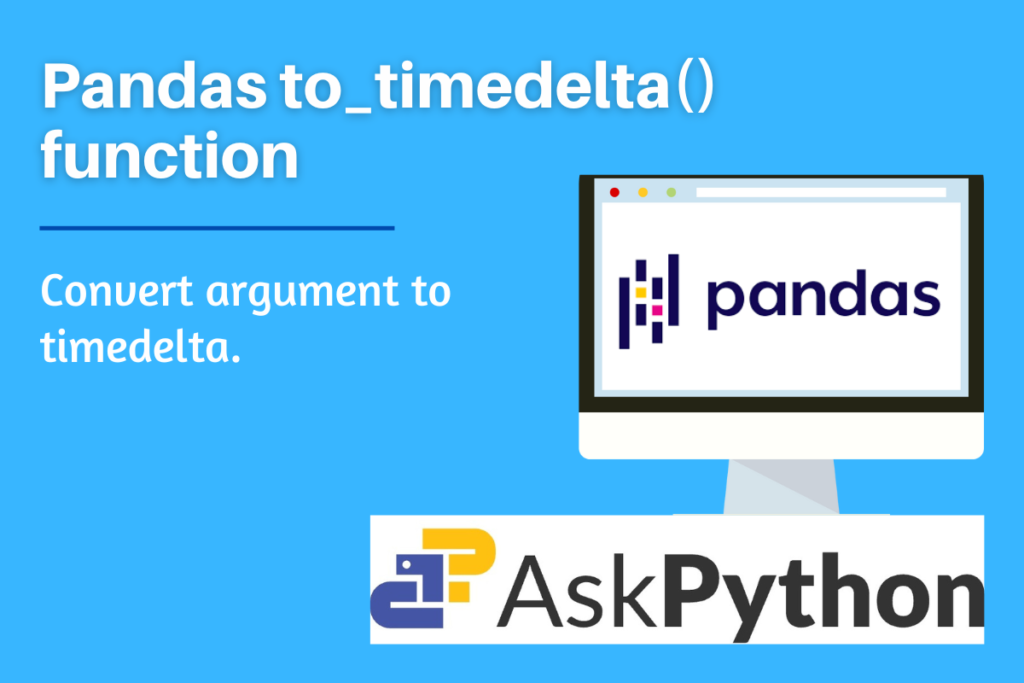# Pandas to_timedelta – Convert argument to timedelta.Let’s attempt to learn one of the general functions of the Pandas package, the `to_timedelta()` function, in this post. The Pandas software package for the Python programming language is used to manipulate and interpret information. Both “Panel Data” and “Python Data Analysis” are meant to refer to as “Pandas.” It provides particular methods and data structures for dealing with mathematical tables and time series.

Also read: Pandas to_datetime – Convert argument to datetime.

## Why is Pandas to_timedelta() used?

By using this method, an accepted timedelta format or value can be converted into a Timedelta type. Absolute time differences represented in different units are known as timedeltas for example- days, hours, minutes, and seconds. In other words, a timedelta object represents a duration, the difference between two dates or times.

The return type for scalar input is timedelta, TimedeltaIndex of timedelta64 data type for list-like input, and Series of timedelta64 data type for Series input.

In a future release, strings with the units “M,” “Y,” and “y” will no longer be supported because they do not accurately represent timedelta values.

## Syntax of Pandas to_timedelta

```pandas.to_timedelta(arg, unit=None, errors='raise')
```
• arg: string list-like, Series, Required
• The data needs to be transformed into a timedelta.
• unit: string, optional
• Indicates the arg’s unit for a numeric arg.
• errors: {‘ignore’, ‘raise’, ‘coerce’}, default ‘raise’, optional
• Invalid parsing will cause an exception if “raise” is set.
• Invalid parsing will be set as NaT if “coerce” is selected.
• Invalid parsing will return the input if ignore is selected.

The unit for argument can be as follows. (the default is set to ‘ns’.

• ‘W’ for a week.
• ‘days’ or ‘day’ or just D
• ‘hours’ or ‘hour’ or ‘hr’ or just ‘h’
• ‘minute’ or ‘min’ or ‘minutes’ or just ‘m’ or ‘T’
• ‘seconds’ or ‘sec’ or ‘second’ or just ‘S’
• ‘milliseconds’ or ‘millisecond’ or ‘milli’ or ‘millis’ or ‘ms’ or ‘L’
• ‘microseconds’ or ‘microsecond’ or ‘micro’ or ‘micros’ ‘us’ or ‘U’
• ‘nanoseconds’ or ‘nano’ or ‘nanos’ or ‘nanosecond’ or ‘ns’ or ‘N’

Note: For string inputs, the precision of the duration is reduced to nanoseconds if the precision is more than that.

## Implementing Pandas to_timedelta() with Examples

Make sure to import the Pandas package in your IDE before implementing the function. To do so, run the following code line first.

```import pandas as pd
```

### Example 1: Passing only argument parameter

```#String Input
x = ("12 days 48 hours")
x_out = pd.to_timedelta(x)

y = ("2 days, 35 min, 4 hours")
y_out = pd.to_timedelta(y)

print(x_out, "\n", y_out)
```
```#list-like / series input
x = ("540 sec", "1.23.177 us", "5 days 01:02:03.00004")
x_out = pd.to_timedelta(x)

y = pd.Series(["20:33:41","40 min","333 ns"])
y_out = pd.to_timedelta(y)

print(x_out, "\n\n", y_out)
```

## Example 2: Passing unit parameter

Note: unit must not be specified if the input is/contains a string, it will through ValueError

To provide an argument, the `arange()` function of the NumPy package is used. (Make sure to import the NumPy package beforehand)

```import numpy as np

#using arange function of NumPy Package
x = pd.to_timedelta(np.arange(3), unit="minutes")
print(x)

y = pd.to_timedelta(np.arange(5,15,2), unit="days")
print("\n", y)
```

## Example 3: Passing Errors parameter

```#Invalid parsing will cause an exception if "raise" is set.
x = ("20 days", "40 hours 65 min", "6 weeks")
pd.to_timedelta(x, errors="raise")
```
```#Invalid parsing will be set as NaT if "coerce" is selected.
x = ("20 days", "40 hours 65 min", "6 weeks")
pd.to_timedelta(x, errors="coerce")
```
```#Invalid parsing will return the input if ignore is selected.